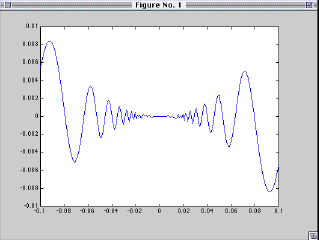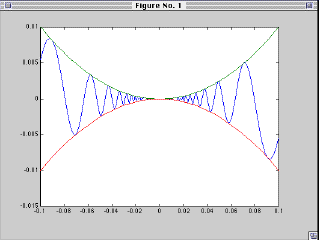# 2D Plotting

Plotting y=f(x) on the interval [-.1,.1]:
First create a vector of (e.g.) 200 equally spaces values between -.1 and .1.
x1=linspace(-.1,.1,200);
Suppose f(x) is an algebraic expression of standard functions such as f(x)= x^2*sin(1/x).
Then the following computes the y values from the x values.
y1 = x1 .^ 2 .* sin(1 ./ x1);
Now put up the plot:
plot(x1,y1)Adding Additional Graphs
can be accomplished by
x2=linspace(-.1,.1,50);
(Note that this function oscillates less, so we take a coarser grid with only 50 points.
y2 = x2 .^2
y3 = -x2 .^2
plot(x1,y1,x2,y2,x2,y3)Last Update: September 17, 1998